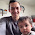## Monday, April 7, 2014

### GTO Brainteaser #1 Solution

Today I'll be going over the solution to the Rock Paper Scissors brain teaser that I posted here: http://blog.gtorangebuilder.com/2014/04/gto-brain-teaser-1-exploitation-and.html and talking a bit about how it relates to poker.

The main problem is solved in the video below, which also has a nice introduction to basic game theory concepts.

### Bonus Solution

I'm going to be using a lot of the terminology and techniques that I introduced in the solution video above, so if you haven't watched it already please check it out here before continuing.

To solve the bonus problem we have to start at the end of the game tree and work our way back to the start via Backwards Induction.

The basic idea of backwards induction is simple, we solve the 2nd round, and then solve the first round by assuming that the payoff for going into the second round in various game states is the equilibrium payoff of the 2nd round in isolation.  An important and related concept is the notion of a subgame perfect equalibrium.

In this case the 2nd round is very easy to solve.  If our opponent did not play rock in the 1st round then they must play it in the 2nd round, and this is known to both players, so they are guaranteed to lose.  If they did play rock in the 1st round, then the second round is just a regular round of rock paper scissors, and the equilibrium payoff in the round is 0 for both players (In the nash equilibrium, both players each play 1/3 rock, 1/3 paper, 1/3 scissors) .

We'll also assume that Player 1 should be able to profit from the game.

We can rule out any pure strategy play for Player 1 by noting that if Player 1 plays a pure strategy in round 1, then his opponents best response will guarantee that he loses in round 1, which means at best he will break even overall.

We can also say that any pure strategy for Player 2 would at best earn -\$50 per round, which is what they get for always playing rock in round 1 and then playing the nash equilibrium of the regular rock paper scissors sub game in round 2.

So assuming player 2 can average better than -\$50 by mixing, both players should play mixed strategies.

We can write out the expected value of our options very quite simply.

EV[P1] = 50 (r2 - s2) + 50 *  (1 - r2)
EV[S1] = 50 (p2 - r2) + 50 * (1 - r2)
EV[R1] = 50 (s2 - p2) + 50 *  (1 - r2)

Here r2, p2, and s2 are the probabilities with which Player 2 plays rock, paper and scissors respectively, and EV[P1] is the expected value of player 1 playing paper.

We can note immediately that if we subtract the second round EVs, which are represented by the 50 * (1 - r2) term from each equation, this makes the equations symmetric in r2, p2,  s2. Thus the solution to the indifference equations must be symmetric.

If we note that r2 + p2 +  s2  = 1, the only symmetric solution is that they are all 1/3.

So Player 2 plays rock, paper and scissors each 1/3.  If we plug those values in to Player 1's EV equations we see that he profits by \$33.33 per round.

For player 2, if we note that playing anything other than rock loses us \$50 in round 2, our EV equations are:

EV[P2] = 50 (r1 - s1) - 50
EV[S2] = 50 (p1 - r1) - 50
EV[R2] = 50 (s1 - p1) - 0

We know that Player 2 is playing all 3 options so indifference conditions require the EVs are all the same. Furthermore, we know that each of those EVs = \$-33.33 since the game is zero-sum.

You can solve these by hand or use something like wolfram alpha, but its easy to check that r1 = 1/3, p1 = 2/3,  s1 = 0 solves all 3 equations properly (it makes them all equal to -\$33.33).

This means that Player 1 plays the same strategy in both the main problem and the bonus problem, but that Player 2's disadvantage is twice as large in the bonus.

Edit:

Note that because P1 is never actually playing scissors, the indifference condition on EV[s1] need not actually hold.  Because of this there can be multiple equilibrium solutions to this game so long as EV[P1] = EV[R1] as h7r so helpfully noted in the comments.

The EV for both players is the same regardless of which equilibrium is played because that is true for all zero sum two player games.

1.That's a nice and clean math.

2.This comment has been removed by the author.

3.I'm loving these brainteasers, really hope to see more of them.

One thing though that I'm a bit confused about: in the bonus puzzle solution when we solve for player 1's EVs, why is the second round EV represented by 50 * r2? Should it not be 50 * (1 - r2) since we only auto-win the second round if player 2 *doesn't* play rock? It's also inconsistent with one of the following paragraphs saying that, if we plug r2=p2=s2=1/3 in we get an EV of \$33.33... which we don't since it simplifies to 50 * 1/3 = \$16.67. Seems like an error to me...

1.You are definitely correct, that is an error, sorry about that. It doesn't effect the solution, and I've corrected it above, thanks for pointing it out!

Glad you like the brainteasers :)